# Linear Arrangements Questions for CMAT PDF

0
215

Here you can download CMAT 2022 – important Linear Arrangement Questions PDF by Cracku. Very Important Linear Arrangement Questions for CMAT 2022 based on asked questions in previous exam papers. These questions will help your CMAT preparation. So kindly download the PDF for reference and do more practice.

Take Free CMAT mock tests here

Instructions

Study the following information carefully and answer the questions given below :

Eight persons – J, K, L, M, W, X, Y and Z – are standing in a straight line, but not necessarily in the same order. Some of them are facing north while some others are facing south. J is standing at the fourth position to the right of X. X is standing at one of the extreme ends of the line. Both the immediate neighbours of J face north. M is standing at the third position to the right of J. M is facing the same direction as that of J. There is only one person between M and L. L is standing at the third position to the right of Y. Z is standing to the immediate left of L. K is not facing north. Z is facing the same direction as that of W. K is not standing at any of the extreme ends of the line.

Question 1: Who among the following is standing at one of the ends excluding X ?

a) Z

b) M

c) W

d) Y

e) L

Question 2: Four of the following five are alike in a certain way based on the above arrangement and hence form a group. Which is the one that does not belong to the group ?

a) M

b) J

c) L

d) Y

e) X

Question 3: Who among the following is to the immediate left of W ?

a) K

b) Y

c) L

d) J

e) None of these

Question 4: How many persons are standing exactly between Y and Z ?

a) Three

b) Four

c) Two

d) Five

e) None of these

Question 5: Who among the following are facing South ?

a) M, J, Y

b) J, K, M, X

c) J. K, M, X, Y

d) J, M, X, Y

e) K, M, X, Y

Instructions

Study the following information carefully and answer the questions given below :

Ten persons are sitting in two parallel rows containing five people each, in such a way that there is equal distance between adjacent persons. In row-1, A, B, C, D and E are seated (but not necessarily in the same order) and all of them are facing north. In row-2, L, M, N, O and P are seated (but not necessarily in the same order) and all of them are facing south. Therefore, in the given seating arrangement each member seated in a row faces another member of the other row. B sits second to the right of D. The person facing B sits to the immediate left of N. L sits second to the right of N. Only two persons sit between L and P. E is not an immediate neighbour of D. O does not face E. C neither faces N nor sits at an extreme end of the line.

Question 6: What is the position of C with respect to B?

a) Second to the left

b) Third to the left

c) Immediate left

d) Immediate right

e) Second to the right

Question 7: Four of the following five are alike in a certain way based on the given arrangement and hence form a group. Which is the one that does not belong to the group?

a) NO

b) CE

c) AB

d) PO

e) ML

Question 8: Who amongst the following is facing M?

a) E

b) A

c) D

d) B

e) C

Question 9: Who amongst the following is facing A?

a) L

b) M

c) 0

d) P

e) N

Question 10: Which of the following statements is TRUE regarding 0?

a) L sits to the immediate left of 0.

b) Only three persons sit between P and 0.

c) 0 sits exactly in the middle of the row.

d) 0 faces one of the immediate neighbours of B.

e) None of the given statements is true

X is at one of the ends. J is fourth to the right of X.

Now this presents 2 scenarios, one where X is facing north and the other where X is facing south.

Considering the scenario where X is facing North :-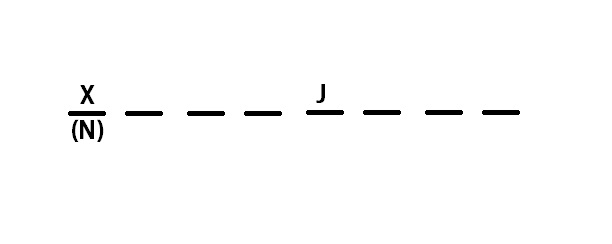Each of the neighbors of J are facing North.
Now J can be facing either north or south. Lets first consider the case when J is facing north.
Now M is facing in the same direction as J and is standing third to the right of J. L is one place away from M. L is also at the third position from the right of Y, thus :-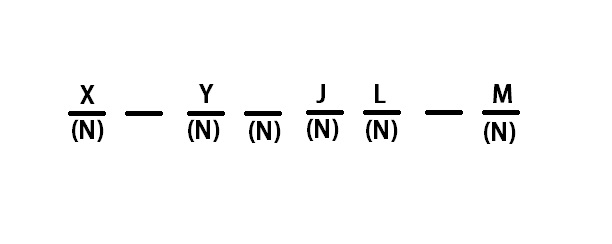Now Z has to stand to the immediate left of L, hence this case is rejected.

Now lets consider the case where J is facing south :-
Again M is facing in the same direction as J and is standing third to the right of J. L is one place away from M. L is also at the third position from the right of Y. Also Z is standing to the immediate left of L. Thus :-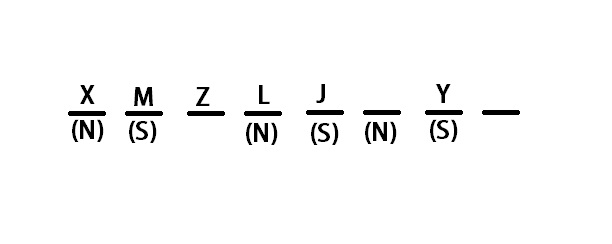Now since K is neither facing north, nor standing at the end, this case too is rejected.

Considering the case where X is facing south :-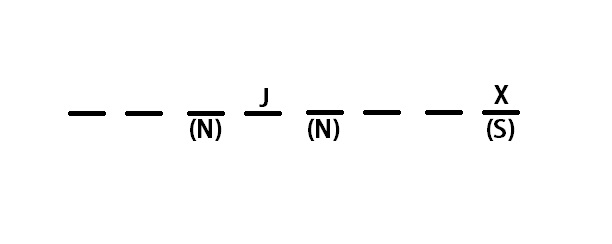Again J can either face North or South.
Considering the case when J is facing North :-
M is facing in the same direction as J and is standing third to the right of J. L is one place away from M.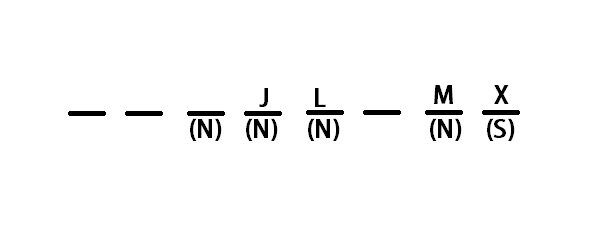In this case, Z can’t stand to the left of L. Hence, this case is rejected.

The only remaining possibility is that J is facing South.
Following all the conditions, the final arrangement looks like :-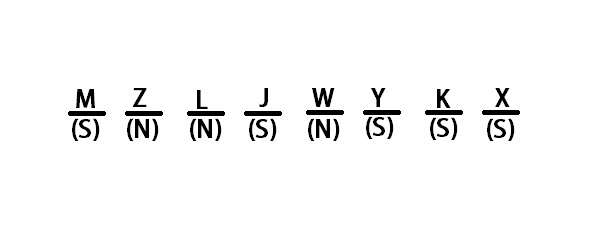M is standing at one of the ends.

X is at one of the ends. J is fourth to the right of X.

Now this presents 2 scenarios, one where X is facing north and the other where X is facing south.

Considering the scenario where X is facing North :-Each of the neighbors of J are facing North.
Now J can be facing either north or south. Lets first consider the case when J is facing north.
Now M is facing in the same direction as J and is standing third to the right of J. L is one place away from M. L is also at the third position from the right of Y, thus :-Now Z has to stand to the immediate left of L, hence this case is rejected.

Now lets consider the case where J is facing south :-
Again M is facing in the same direction as J and is standing third to the right of J. L is one place away from M. L is also at the third position from the right of Y. Also Z is standing to the immediate left of L. Thus :-Now since K is neither facing north, nor standing at the end, this case too is rejected.

Considering the case where X is facing south :-Again J can either face North or South.
Considering the case when J is facing North :-
M is facing in the same direction as J and is standing third to the right of J. L is one place away from M.In this case, Z can’t stand to the left of L. Hence, this case is rejected.

The only remaining possibility is that J is facing South.
Following all the conditions, the final arrangement looks like :-M, J, Y and X face South. L is the only one among the given options facing North.

X is at one of the ends. J is fourth to the right of X.

Now this presents 2 scenarios, one where X is facing north and the other where X is facing south.

Considering the scenario where X is facing North :-Each of the neighbors of J are facing North.
Now J can be facing either north or south. Lets first consider the case when J is facing north.
Now M is facing in the same direction as J and is standing third to the right of J. L is one place away from M. L is also at the third position from the right of Y, thus :-Now Z has to stand to the immediate left of L, hence this case is rejected.

Now lets consider the case where J is facing south :-
Again M is facing in the same direction as J and is standing third to the right of J. L is one place away from M. L is also at the third position from the right of Y. Also Z is standing to the immediate left of L. Thus :-Now since K is neither facing north, nor standing at the end, this case too is rejected.

Considering the case where X is facing south :-Again J can either face North or South.
Considering the case when J is facing North :-
M is facing in the same direction as J and is standing third to the right of J. L is one place away from M.In this case, Z can’t stand to the left of L. Hence, this case is rejected.

The only remaining possibility is that J is facing South.
Following all the conditions, the final arrangement looks like :-J is standing to the immediate left of W.

X is at one of the ends. J is fourth to the right of X.

Now this presents 2 scenarios, one where X is facing north and the other where X is facing south.

Considering the scenario where X is facing North :-Each of the neighbors of J are facing North.
Now J can be facing either north or south. Lets first consider the case when J is facing north.
Now M is facing in the same direction as J and is standing third to the right of J. L is one place away from M. L is also at the third position from the right of Y, thus :-Now Z has to stand to the immediate left of L, hence this case is rejected.

Now lets consider the case where J is facing south :-
Again M is facing in the same direction as J and is standing third to the right of J. L is one place away from M. L is also at the third position from the right of Y. Also Z is standing to the immediate left of L. Thus :-Now since K is neither facing north, nor standing at the end, this case too is rejected.

Considering the case where X is facing south :-Again J can either face North or South.
Considering the case when J is facing North :-
M is facing in the same direction as J and is standing third to the right of J. L is one place away from M.In this case, Z can’t stand to the left of L. Hence, this case is rejected.

The only remaining possibility is that J is facing South.
Following all the conditions, the final arrangement looks like :-3 people are standing between Y and Z.

X is at one of the ends. J is fourth to the right of X.
Now this presents 2 scenarios, one where X is facing north and the other where X is facing south.

Considering the scenario where X is facing North :-Each of the neighbors of J are facing North.
Now J can be facing either north or south. Lets first consider the case when J is facing north.
Now M is facing in the same direction as J and is standing third to the right of J. L is one place away from M. L is also at the third position from the right of Y, thus :-Now Z has to stand to the immediate left of L, hence this case is rejected.

Now lets consider the case where J is facing south :-
Again M is facing in the same direction as J and is standing third to the right of J. L is one place away from M. L is also at the third position from the right of Y. Also Z is standing to the immediate left of L. Thus :-Now since K is neither facing north, nor standing at the end, this case too is rejected.

Considering the case where X is facing south :-Again J can either face North or South.
Considering the case when J is facing North :-
M is facing in the same direction as J and is standing third to the right of J. L is one place away from M.In this case, Z can’t stand to the left of L. Hence, this case is rejected.

The only remaining possibility is that J is facing South.
Following all the conditions, the final arrangement looks like :-For this question, M,J,Y,K and X are facing south.

B sits second to the right of D and The person facing B sits to the immediate left of N :-

[ _ ] [ N ] [ _ ]
[ D ] [ _ ] [ B ]

L sits second to the right of N. Only two persons sit between L and P.
[ L ] [ _ ] [ N ] [ P ]
[ _ ] [ D ] [ _ ] [ B ]

E is not an immediate neighbour of D. 0 does not face E.
[ M ] [ L ][ O ] [ N ] [ P ] Or [ L ][ O ] [ N ] [ P ] [ M ]
[ E ] [ __ ][ D ] [ _ ] [ B ] Or [ _ ][ D ] [ __ ] [ B ] [ E ]

C neither faces N nor sits at an extreme end of the line
[ M ] [ L ][ O ] [ N ] [ P ]
[ E ] [ C ][ D ] [ A ] [ B ]

C sits third to the left of B.

B sits second to the right of D and The person facing B sits to the immediate left of N :-

[ _ ] [ N ] [ _ ]
[ D ] [ _ ] [ B ]

L sits second to the right of N. Only two persons sit between L and P.
[ L ] [ _ ] [ N ] [ P ]
[ _ ] [ D ] [ _ ] [ B ]

E is not an immediate neighbour of D. 0 does not face E.
[ M ] [ L ][ O ] [ N ] [ P ] Or [ L ][ O ] [ N ] [ P ] [ M ]
[ E ] [ __ ][ D ] [ _ ] [ B ] Or [ _ ][ D ] [ __ ] [ B ] [ E ]

C neither faces N nor sits at an extreme end of the line
[ M ] [ L ][ O ] [ N ] [ P ]
[ E ] [ C ][ D ] [ A ] [ B ]

All the options except PO present a couple of neighbors.

B sits second to the right of D and The person facing B sits to the immediate left of N :-

[ _ ] [ N ] [ _ ]
[ D ] [ _ ] [ B ]

L sits second to the right of N. Only two persons sit between L and P.
[ L ] [ _ ] [ N ] [ P ]
[ _ ] [ D ] [ _ ] [ B ]

E is not an immediate neighbour of D. 0 does not face E.
[ M ] [ L ][ O ] [ N ] [ P ] Or [ L ][ O ] [ N ] [ P ] [ M ]
[ E ] [ __ ][ D ] [ _ ] [ B ] Or [ _ ][ D ] [ __ ] [ B ] [ E ]

C neither faces N nor sits at an extreme end of the line
[ M ] [ L ][ O ] [ N ] [ P ]
[ E ] [ C ][ D ] [ A ] [ B ]

E is facing M.

B sits second to the right of D and The person facing B sits to the immediate left of N :-
[ _ ] [ N ] [ _ ]
[ D ] [ _ ] [ B ]

L sits second to the right of N. Only two persons sit between L and P.
[ L ] [ _ ] [ N ] [ P ]
[ _ ] [ D ] [ _ ] [ B ]

E is not an immediate neighbour of D. 0 does not face E.
[ M ] [ L ][ O ] [ N ] [ P ] Or [ L ][ O ] [ N ] [ P ] [ M ]
[ E ] [ __ ][ D ] [ _ ] [ B ] Or [ _ ][ D ] [ __ ] [ B ] [ E ]

C neither faces N nor sits at an extreme end of the line
[ M ] [ L ][ O ] [ N ] [ P ]
[ E ] [ C ][ D ] [ A ] [ B ]

N sits opposite A.

B sits second to the right of D and The person facing B sits to the immediate left of N :-
[ _ ] [ N ] [ _ ]
[ D ] [ _ ] [ B ]

L sits second to the right of N. Only two persons sit between L and P.
[ L ] [ _ ] [ N ] [ P ]
[ _ ] [ D ] [ _ ] [ B ]

E is not an immediate neighbour of D. 0 does not face E.
[ M ] [ L ][ O ] [ N ] [ P ] Or [ L ][ O ] [ N ] [ P ] [ M ]
[ E ] [ __ ][ D ] [ _ ] [ B ] Or [ _ ][ D ] [ __ ] [ B ] [ E ]

C neither faces N nor sits at an extreme end of the line
[ M ] [ L ][ O ] [ N ] [ P ]
[ E ] [ C ][ D ] [ A ] [ B ]

As apparent, O sits in the exact middle.